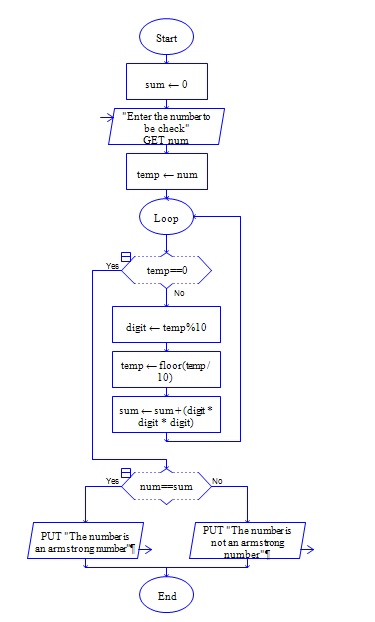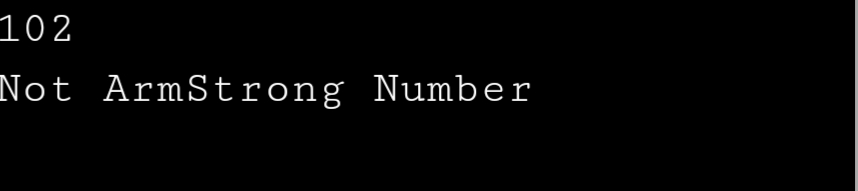# C Program to check whether given Integer is Armstrong or Not

Sample Input 1 :

370

Sample Output 1 :

ArmStrong (3*3*3 + 7*7*7 + 0=370)

Note: Cube digits for 3 digit numbers, square digits for two digit number and soon.

#### Flow Chart Design#### Program or Solution

``` #include<stdio.h> int main() { int n,num,count,rem,i,m,k=0; scanf("%d",&num); n=num; while(num!=0) { num/=10; count++; } num=n; while(num!=0) { rem=num%10; m=1; for(i=1;i<=count;i++) { m*=rem; } k+=m; num=num/10; } if(n==k) { printf("Armstrong Number"); } else { printf("Not ArmStrong Number"); } return 0; } ```

#### Output#### Program Explanation

refer video tutorial